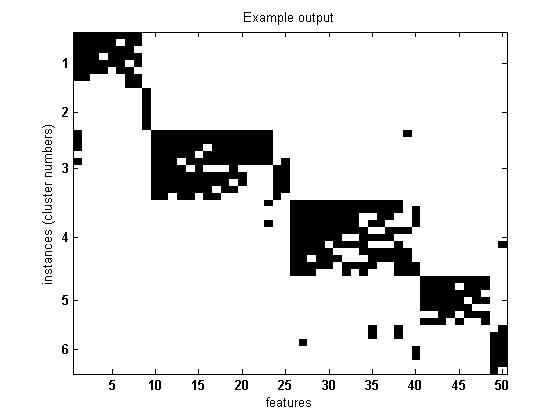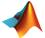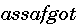Spectral CoClustering (Biclustering) Matlab implementation

The following Matlab m-files implement a bipartite spectral graph partitioning algorithm of (Dhillon, 2001).
The algorithm was designed to cocluster (bicluster) sparse binary co-occurrences of documents and words.
We augmented the algorithm to provide better images by applying single linkage hierarchical algorithm for each produced bicluster individually.
We then sort each bicluster according to the hierarchical clustering ordering, which gives cleaner look to less homogenous clusters.

The software comes with no guarantees.

Example:

Example output (k=6 clusters, using the PlotCoClustering.m wrapper to display only cluster numbers):The following m-files are required (returning visitors - please pay attention to the modified function output):

 SpectralCoClustering.m (main function)ArrangeData2Clusters.m (called from SpectralCoClustering.m)Tips & clarifications:

• The algorithm uses the kmeans algorithm and thus may give different outputs for different runs.
• Although Dhillon introduced this algorithm as applicable on all sort of matrices,
according to my experience, the algorithm works best when the number of possible values is low (preferably binary or ternary).
• The algorithm outputs errors when the matrix contains empty rows or columns.
• Make sure that the matrix is not too sparse, so clusters will have reasonable support set.
Instances with only one or two features that have values or alternatively,
features which have values for only very few instances should be eliminated.
If you need to remove too sparse rows and columns, you can use the following file:
• If you want to display the instances names on the y-axis, input them as a cell array in the 4th parameter.
• If you want to display the cluster numbers on the y-axis for each instance, input 1 in the 4th parameter instead.
For outputting the cluster numbers only for cluster centers, please use the following wrapper: